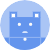search
Search
Guest 0reps
Thanks for the thanks!
close
chevron_left Type Conversion
Cancel
Post
account_circle
Profile
exit_to_app
Sign out
search
keyboard_voice
close
Searching Tips
Search for a recipe:
"Creating a table in MySQL"
Search for an API documentation: "@append"
Search for code: "!dataframe"
Apply a tag filter: "#python"
Useful Shortcuts
/ to open search panel
Esc to close search panel
to navigate between search results
d to clear all current filters
Enter to expand content previewDoc SearchCode Search BetaSORRY NOTHING FOUND!
mic
Start speaking...Voice search is only supported in Safari and Chrome.
Shrink
Navigate to
A
A
brightness_medium
share
arrow_backShareTwitterFacebook
chevron_left Type Conversion
0
thumb_down
0
chat_bubble_outline
0
auto_stories new
settings

# Pandas DataFrame | to_numpy method

Programming
chevron_right
Python
chevron_right
Pandas
chevron_right
Documentation
chevron_right
DataFrame
chevron_right
Type Conversion
schedule Jul 1, 2022
Last updated
local_offer PythonPandas
Tags
expand_more

Pandas `DataFrame.to_numpy(~)` method returns the values of the DataFrame as a 2D NumPy array.

# Parameters

1. `dtype`link | `string` or `type` | `optional`

The desired data type of the returned NumPy array. By default, the data type will be the common type of the array's values. See examples below for clarification.

2. `copy`link | `boolean` | `optional`

• If `True`, then a new NumPy array is created. Modifying this array would not affect the source DataFrame and vice versa.

• If `False`, then a reference to the DataFrame's NumPy array representation is returned. This means that if you modify the array, then the original DataFrame will also be modified, and vice versa.

By default `copy=False`.

# Return Value

A `Numpy array` holding all the values of the source DataFrame.

# Examples

## Obtaining the NumPy Array representation

Consider the following DataFrame:

``` df = pd.DataFrame({"A":[1,2], "B":[3,4]})df    A  B0  1  31  2  4 ```

To get the values of `df` as a NumPy array:

``` df.to_numpy() array([[1, 3],       [2, 4]]) ```

## Data type of returned NumPy array

Consider the following DataFrame:

``` df = pd.DataFrame({"A":[1,2], "B":[3.0,4.0]})df A B0  1  3.01  2  4.0 ```

Here, column `A` is of type `int`, while column `B` is of type `float`.

The limitation with NumPy arrays is that all their values must be of one type. Since our `df` has two types, the `to_numpy(~)` method will opt to use `float` as `int` can be represented using `float`:

``` df.to_numpy().dtype dtype('float64') ```

## Creating a new copy

Consider the following DataFrame:

``` df = pd.DataFrame({"A":[1,2], "B":[3,4]})df A B0  1  31  2  4 ```

To create a new NumPy array, set `copy=True`. In the code snippet below, we modify the first value of the array and check to see whether the source DataFrame, `df`, has been modified:

``` arr = df.to_numpy(copy=True)arr[0,0] = 5df    A  B0  1  31  2  4 ```

Notice how the first value of the DataFrame (`1`) is left intact since `arr` is a copy of `df`.

mail